# Lecture 10 Relational Algebra 1 Relational Algebra A

• Slides: 23
Download presentation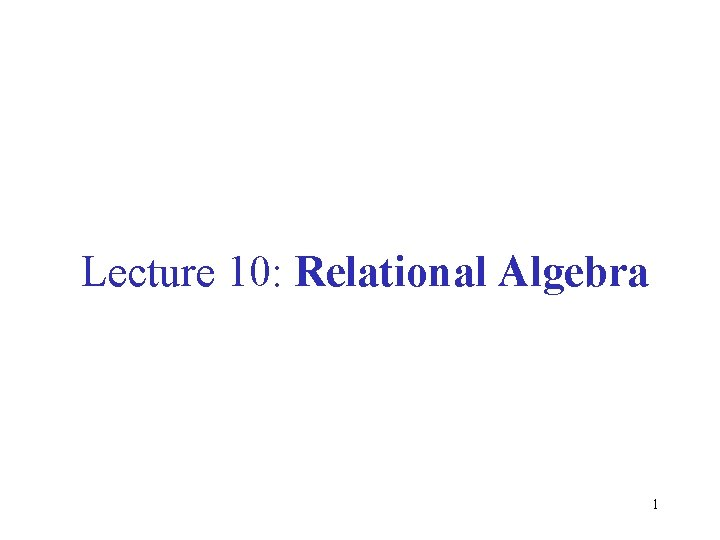Lecture 10: Relational Algebra 1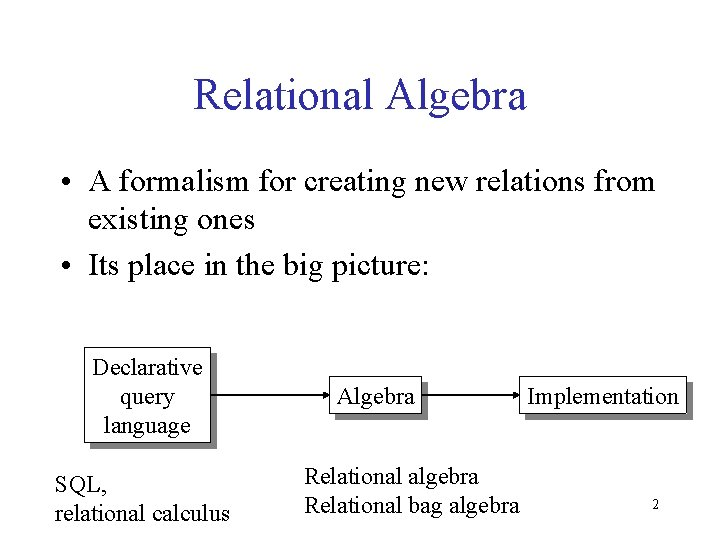Relational Algebra • A formalism for creating new relations from existing ones • Its place in the big picture: Declarative query language SQL, relational calculus Algebra Relational algebra Relational bag algebra Implementation 2Relational Algebra • Five operators: – – – Union: Difference: Selection: s Projection: P Cartesian Product: • Derived or auxiliary operators: – Intersection, complement – Joins (natural, equi-join, theta join, semi-join) – Renaming: r 31. Union and 2. Difference • R 1 R 2 • Example: – Active. Employees Retired. Employees • R 1 – R 2 • Example: – All. Employees – Retired. Employees 4What about Intersection ? • • It is a derived operator R 1 R 2 = R 1 – (R 1 – R 2) Also expressed as a join (will see later) Example – Unionized. Employees Retired. Employees 5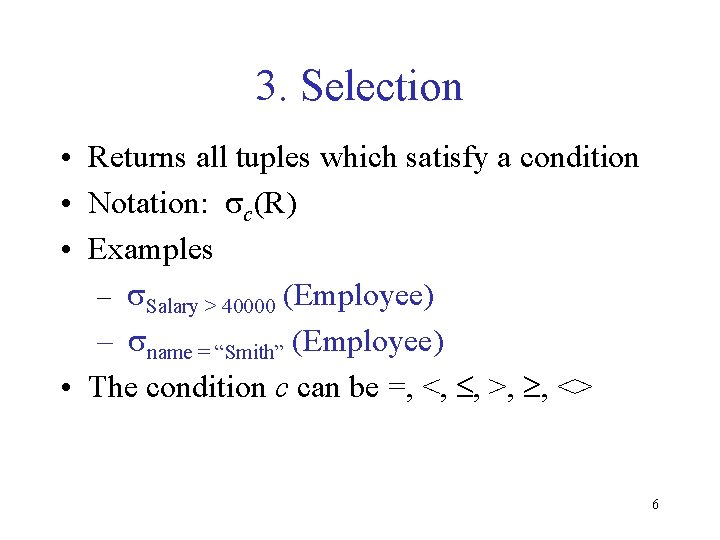3. Selection • Returns all tuples which satisfy a condition • Notation: sc(R) • Examples – s. Salary > 40000 (Employee) – sname = “Smith” (Employee) • The condition c can be =, <, , >, , <> 6Find all employees with salary more than \$40, 000. s. Salary > 40000 (Employee) 7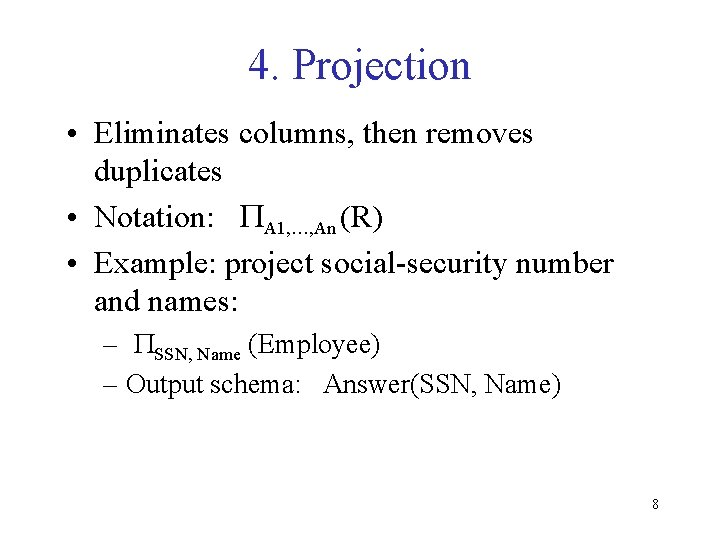4. Projection • Eliminates columns, then removes duplicates • Notation: PA 1, …, An (R) • Example: project social-security number and names: – PSSN, Name (Employee) – Output schema: Answer(SSN, Name) 8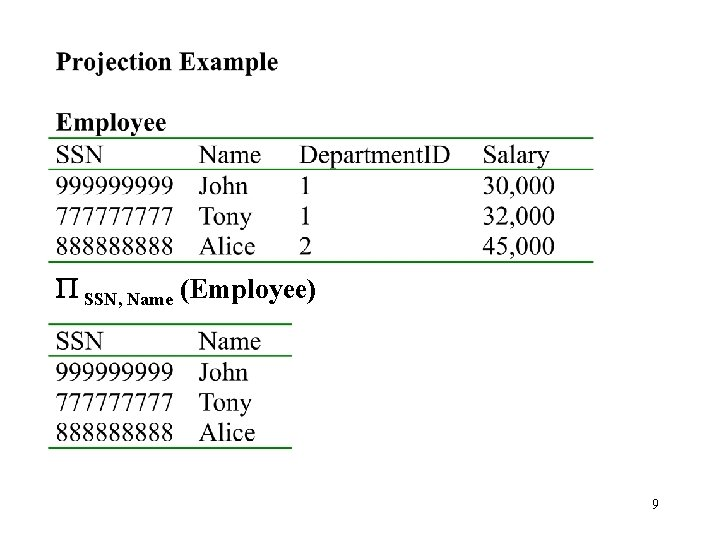P SSN, Name (Employee) 95. Cartesian Product • Each tuple in R 1 with each tuple in R 2 • Notation: R 1 R 2 • Example: – Employee Dependents • Very rare in practice; mainly used to express joins 1011Relational Algebra • Five operators: – – – Union: Difference: Selection: s Projection: P Cartesian Product: • Derived or auxiliary operators: – Intersection, complement – Joins (natural, equi-join, theta join, semi-join) – Renaming: r 12Renaming • Changes the schema, not the instance • Notation: r B 1, …, Bn (R) • Example: – r. Given. Name, Soc. Sec. No (Employee) – Output schema: Answer(Given. Name, Soc. Sec. No) 13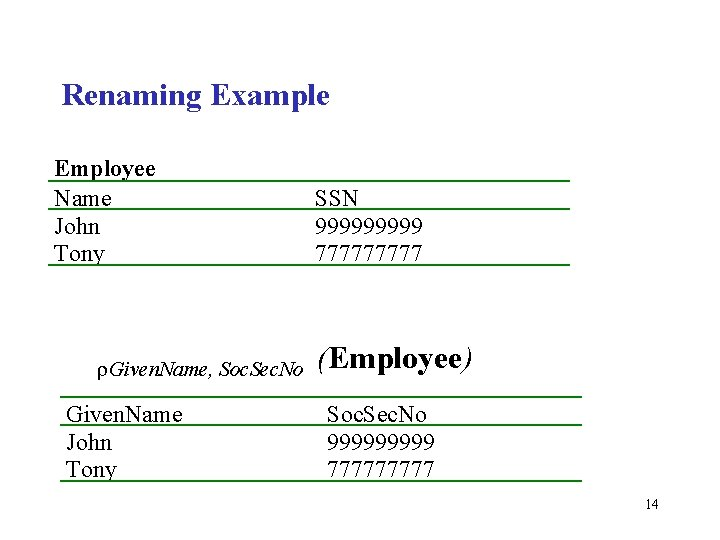Renaming Example Employee Name John Tony r. Given. Name, Soc. Sec. No Given. Name John Tony SSN 99999 77777 (Employee) Soc. Sec. No 99999 77777 14Natural Join • Notation: R 1 ⋈ R 2 • Meaning: R 1 ⋈ R 2 = PA(s. C(R 1 R 2)) • Where: – The selection s. C checks equality of all common attributes – The projection eliminates the duplicate common attributes 15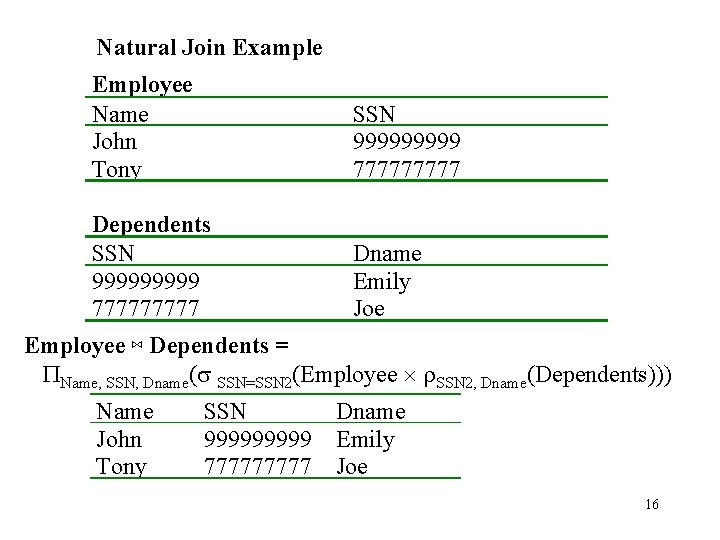Natural Join Example Employee Name John Tony SSN 99999 77777 Dependents SSN 99999 77777 Dname Emily Joe Employee ⋈ Dependents = PName, SSN, Dname(s SSN=SSN 2(Employee r. SSN 2, Dname(Dependents))) Name John Tony SSN 99999 77777 Dname Emily Joe 16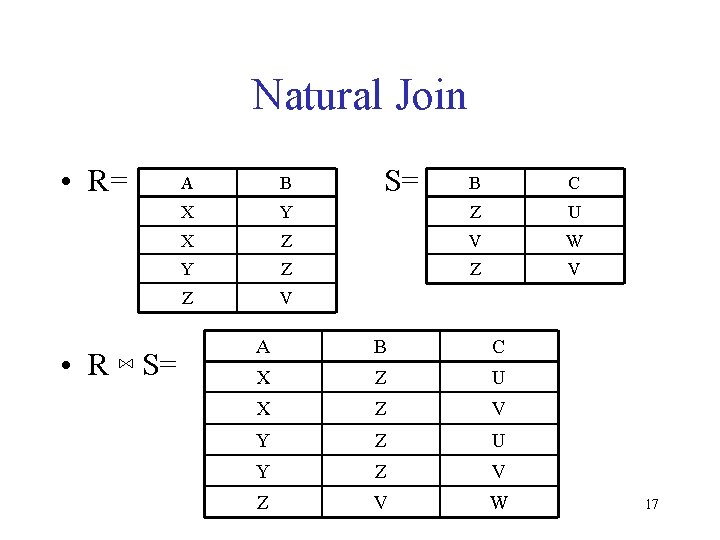Natural Join • R= • R ⋈ S= A B X S= B C Y Z U X Z V W Y Z Z V A B C X Z U X Z V Y Z U Y Z V W 17Natural Join • Given the schemas R(A, B, C, D), S(A, C, E), what is the schema of R ⋈ S ? • Given R(A, B, C), S(D, E), what is R ⋈ S ? • Given R(A, B), S(A, B), what is R ⋈ S ? 18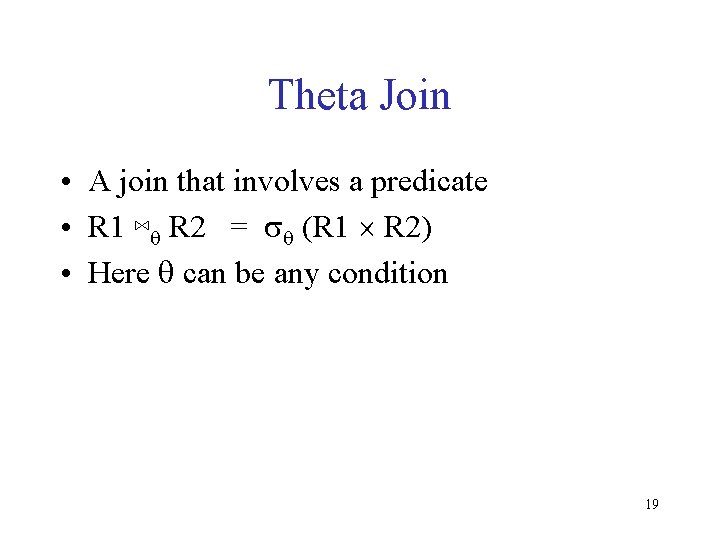Theta Join • A join that involves a predicate • R 1 ⋈q R 2 = sq (R 1 R 2) • Here q can be any condition 19Equi-join • A theta join where q is an equality • R 1 ⋈A=B R 2 = s. A=B (R 1 R 2) • Example: – Employee ⋈SSN=SSN Dependents • Most useful join in practice 20Semijoin • R ⋉ S = PA 1, …, An (R ⋈ S) • Where A 1, …, An are the attributes in R • Example: – Employee ⋉ Dependents 21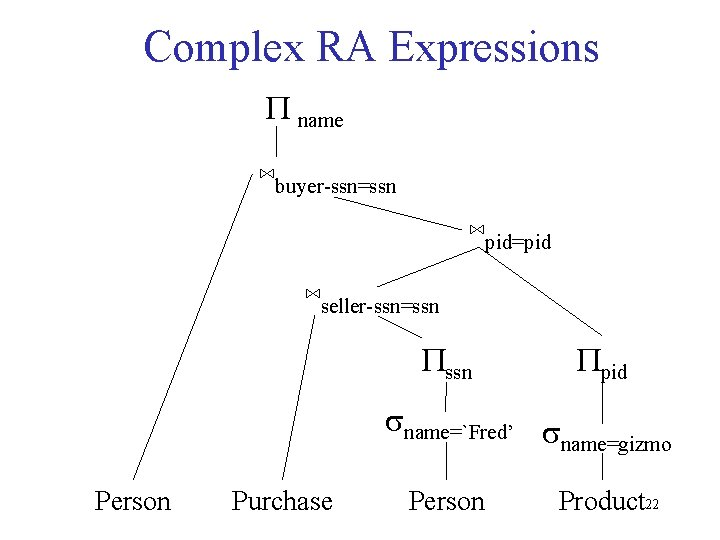Complex RA Expressions P name ⋈buyer-ssn=ssn ⋈pid=pid ⋈seller-ssn=ssn Ppid sname=`Fred’ s name=gizmo Person Purchase Person Product 22Operations on Bags A bag = a set with repeated elements All operations need to be defined carefully on bags • {a, b, b, c} {a, b, b, b, e, f, f}={a, a, b, b, b, c, e, f, f} • {a, b, b, b, c, c} – {b, c, c, c, d} = {a, b, b} • s. C(R): preserve the number of occurrences • PA(R): no duplicate elimination • Cartesian product, join: no duplicate elimination • New operator for Duplicate Elimination Relational Engines work on bags, not sets ! Reading assignment: 5. 1 -5. 2 23